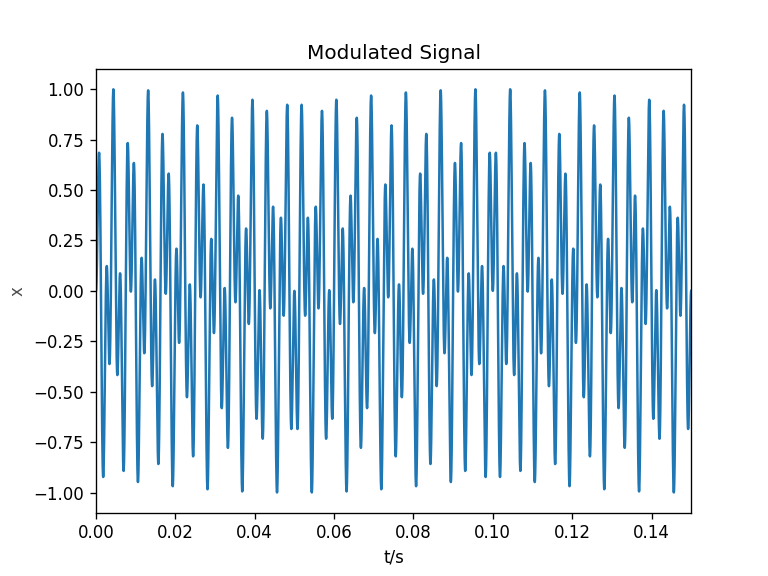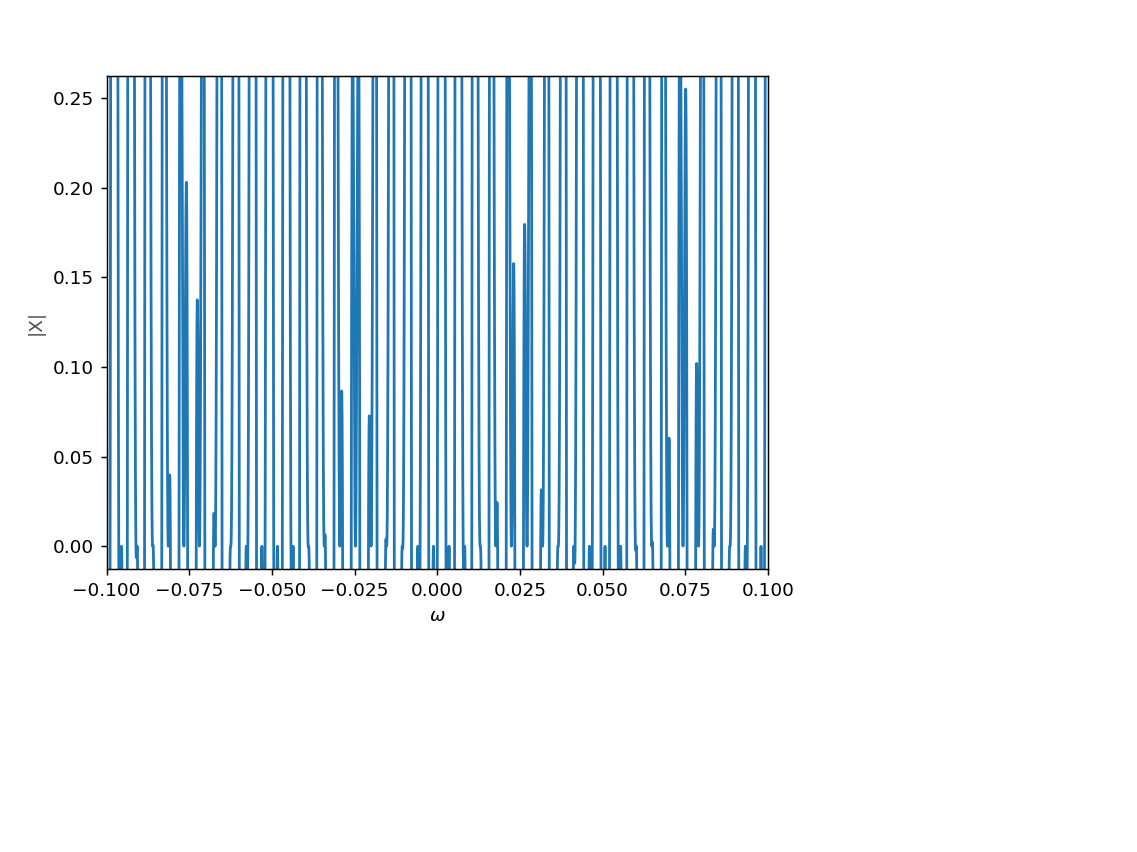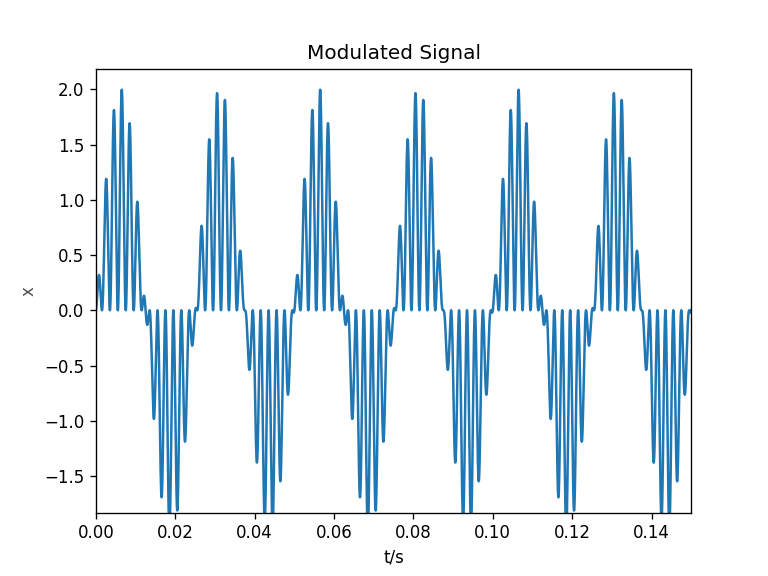# Faust: MIDI

## Using MIDI CC

Using MIDI in Faust requires only minor additions to the code and compiler arguments. For first steps it can be helpful to control single synth parameters with MIDI controllers. This can be configured via the UI elements. The following example uses MIDI controller number 48 to control the frequency of a sine wave by adding [midi:ctrl 48] to the hslider parameters.

// midi-example.dsp
//
// Control a sine wave frequency with a MIDI controller.
//
// Henrik von Coler
// 2020-05-17

import("stdfaust.lib");

freq = hslider("frequency[midi:ctrl 48]",100,20,1000,0.1) : si.smoo;

process = os.osc(freq) <: _,_ ;


CC 48 has been chosen since it is the first slider on the AKAI APC mini. If the controller numbers for other devices are not known, they can be found using the PD patch reverse_midi.pd.

## Compiling with MIDI

In order to enable the MIDI functions, the compiler needs to be called with an additional flag -midi:



## MIDI on Linux

Faust programs use Jack MIDI, whereas MIDI controllers usually connect via ALSA MIDI. In order to control the synth with an external controller, a bridge is nedded:

$a2jmidi_bridge  The MIDI controller can now connect to the a2j_bridge input, which is then connected to the synth input. # Subtractive: Families ## Subtractive Families Based on the same principle, different families of subtractive synthesizers can be defined - without claim to completeness: • analog modular • analog monophonic • analog polyphonic • virtual analog / analog modeling These sub-groups of subtractive synthesis have different characteristics and are used for different applications. ### Analog Modular The first subtractive synthesizers were large, cupboard-like devices and rather expensive. Although multiple voices are possible, they are designed as monophonic instruments. Two well known and opposite examples are the first models released by Bob Moog on the US east coast and Don Buchla on the west coast. • Moog Synthesizer (1965) - Switched on Bach, Wendy Carlos, 1968 Bob Moog brought the typical VCO-VCA-VCF approach in a rack with a keyboard. It could hence be performed like a typical piano-like instrument. • Buchla 100 (1965) - Silver Apples of the Moon, Morton Subotnick, 1967 Although the Buchla works with slightly different concepts than subtractive synthesis in the Moog synthesizers, it is mentioned in this list. ### Analog Monophonic Analog monophonic synthesizers used the same techniques, yet in a more compact design and not fully modular. This made them more affordable and hence more disseminated and used. Minimoog Model D (1970) The idea of monophonic analog subtractive synthesis is usually linked to the Minimoog.Designed as a fully integrated musical instrument, the Minimoog could be used as a virtous tool for expressive musical performances. Chick Corea's Minimoog solo, starting at 4:20, is a prime example: EMS VCS 3 (1969) The semi-modular EMS allows access to the signal flow in a patching matrix. It is thus more suited for experimemtal sounds, as used by Pink Floyd in Welcome to the Machine for the wobbling machine sounds in the beginning: Roland TB 303 (1982) Although released several years later - and for a different application - the TB303 fits best into this family. The TB 303 was intended to be used as an accompanying instrument for musicians. It has a different interface, a programmable step sequencer.Due to the quirky filters it failed as a bass accompaniment but gave birth to techno and especially acid: ### Analog Polyphonic After the monophonic analog synths of the 70s, which were intended as solo instruments, came the polyphonic ones. Polyphonic analog synthesizers shaped the sound of 80s pop (and especially synth-pop) music with their recognizable sound, often used as pads and harmonic foundation or for bass lines. Yamaha CS-80 (1977) Sequential Circuits Prophet-5 --- Oberheim OBx (1979) Roland Jupiter-8 • 1981 ### Virtual Analog When digital technology was ready, it took over and various synthesizers were released which emulated the principles of subtractive synthesis. These devices were much cheaper and the digital means could provide more voices with better memory options. Virtual analog synthesizers were the backbone of trance development. They lack some of the analog warmth but are tighter in sound. Clavia Nord Lead • 1995 Roland JP-8000 • 1996 The Supersaw Access Virus • 1997 Yamaha AN1x • 1997 # Subtractive: Introduction Subtractive synthesis is probably the most famous and most popular method of sound synthesis. The basic idea is to generate spectra with rich spectral content which are then shaped afterwards by filters. Although the possibilities of subtractive synthesis are quasi-unlimited, especially when combined with other methods, the principle can be exlpained with three groups of functional units: Generators • Oscillators • Noise Generators • ... Modulators • LFO (Low Frequency Oscillators) • Envelopes (ADSR) • ... Manipulators • Filters (VFC) • Attenuators (VCA) • ... [Fig.1] gives an overview how these functional units are arranged in a subtractive synthesizer. Modulators and generators overlap, since they are interchangeable in many aspects.[Fig.1] Functional units in subtractive synthesis. Like with all methods for sound synthesis, the dynamic change of timbre is an essential target for generating the desired sounds. [Fig.2] shows a more specific signal flow which is a typical subtractive synth patch for generating lead or bass sounds. • A VCO is manipulated by a VCF and then attenuated by a VCA. • The VCO has a sawtooth waveform. • The cutoff frequency of the VCF and the amplitude of the VCA are controlled with individual envelopes. • If ENV2 has a faster decay than ENV1, the resulting sound is the typical thump.[Fig.2] Subtractive patch for bass and lead synths, as used in the Faust example. # FM Synthesis: Faust Example The following Faust example is a triggered two-operator FM synth. Both operator frequencies and the modulation index can be adjusted through sliders. Global amplitude and modulation index have individual temporal envelopes with adjustable release times. // fm-simple.dsp // // 2-operator FM synthesis // // - with trigger // - dynamic modulation index // through temporal envelope // // Henrik von Coler // 2020-05-11 import("stdfaust.lib"); ///////////////////////////////////////////////////////// // UI ELEMENTS ///////////////////////////////////////////////////////// trigger = button("Trigger"); f_1 = hslider("OP 1 Frequency",100,0.01,1000,0.1); f_2 = hslider("OP 2 Frequency",100,0.01,1000,0.1); ind_1 = hslider("Modulation Index",0,0,1000,0.1); // a slider for the first release time r1 = hslider("Release 1",0.5,0.01,5,0.01); // a slider for the second release time r2 = hslider("Release 2",0.5,0.01,5,0.01); ///////////////////////////////////////////////////////// // FM Function ///////////////////////////////////////////////////////// am(f1, f2, t1, r1, r2) = gain * os.osc(f1 + (os.osc(f2) * ind_1)* index1) with { gain = en.arfe(0.01, r2, 0,t1); index1 = en.arfe(0.01, r1, 0,t1); }; ///////////////////////////////////////////////////////// // processing ///////////////////////////////////////////////////////// process = am(f_1,f_2, trigger, r1 ,r2) <: _,_;  # AM & Ringmodulation: Faust Examples ## Ringmodulator with Audio Input The Ringmodulator is a simple, characteristic audio effect which has been used in many contextes. There is a large variety of guitar effect pedals based on ringmodulation. Another popular application is alienating voices, as done in vintage SciFi movies. The following example ringmod-input.dsp from the Faust repository modulates an audio input signal with a sine wave of adjustable frequency. // ringmod-input.dsp // // Ringmodulator for audio input // // - fader for controlling modulator frequency // - fader for controlling mix of ringmod // // Henrik von Coler // 2020-05-12 import("stdfaust.lib"); f_m = hslider("Modulator Frequency",100,0.01,1000,0.1); mix = hslider("Modulation Mix",0.5,0,1,0.01); am(x, fm) = (1-mix) * x + mix * x * os.osc(fm); process(x) = am(x,f_m) <: _,_;  ## AM - Ringmod Explorer When used with both sinusoidal carrier and modulator, Ringmodulator an AM become precice means for generating timbres in electronic music contexts. The example am-ringmod.dsp makes the tonal difference between AM and Ringmodulation audible. // am-ringmod.dsp // // Example for amplitude modulation // and ringmodulation. // // - steady sound // - adjustable frequencies // - fader for morphing between am/ringmod // // Henrik von Coler // 2020-05-11 import("stdfaust.lib"); f_x = hslider("Signal Frequency",100,0.01,1000,0.1); f_m = hslider("Modulator Frequency",100,0.01,1000,0.1); m_off = hslider("Modulator Offset",0,0,0.5,0.01); am(fx, fm) = os.osc(fx) * ((1-m_off) * os.osc(fm) + m_off); process = am(f_x,f_m) <: _,_;  # AM & Ringmodulation: Formula & Spectrum ## Amplitude Modulation vs Ringmodulation The basic formula is the same for amplitude modulation and ringmodulation: $$y[n] = x[n] \cdot m[n]$$ However, for ringmodulation the modulation signal is symmetric: $$y[n] = \sin\left(2 \pi f_1 \frac{n}{f_s}\right) \cdot \left(\sin\left[2 \pi f_m \frac{n}{f_s}\right]\right)$$ Whereas for amplitude modulation, the signal ist asymetric: $$y[n] = \sin\left(2 \pi f_1 \frac{n}{f_s}\right) \cdot \left( 1+ \sin\left[2 \pi f_m \frac{n}{f_s}\right]\right)$$ This differnce has an influence on the resulting spectrum and on the sound, as the following examples show. import numpy as np import matplotlib.pyplot as plt %matplotlib notebook from scipy import signal from IPython.display import display, Markdown, clear_output import IPython.display as ipd from ipywidgets import * # define functions for AM and ringmod def am(f1, f2, t): x = np.sin(2*np.pi*f1*t) m = 1+np.sin(2*np.pi*f2*t) y = x*m return(y,m,x) def ringmod(f1, f2, t): x = np.sin(2*np.pi*f1*t) m = np.sin(2*np.pi*f2*t) y = x*m return(y,m,x)  ### AM Spectrum $$Y = DFT(y)$$ The spectrum for amplitude modulation can be calculated as follows: $$Y[k] = \sum_{n=0}^{N-1} y[n] \cdot e^{-j 2 \pi k \frac{n}{N}}$$ $$= \sum_{n=0}^{N-1} \sin\left(2 \pi f_1 \frac{n}{f_s}\right) \cdot \left( 1+ \sin\left[2 \pi f_m \frac{n}{f_s}\right]\right) \cdot e^{-j 2 \pi k \frac{n}{N}}$$ $$=\sum_{n=0}^{N-1} \left( \sin\left(2 \pi f_1 \frac{n}{f_s}\right) + 0.5 \left( \cos\left(2 \pi (f_1 - f_m)\frac{n}{f_s}\right) - \cos\left(2 \pi (f_1 + f_m)\frac{n}{f_s}\right) \right) \right) \cdot e^{-j 2 \pi k \frac{n}{N}}$$ $$= delta[f_1] + 0.5 \delta[f_1 - f_m] + 0.5 \ \delta[f_1 + f_m]$$ AM creates a spectrum with a peak at the carrier frequency and two peaks below and above it. Their position is defined by the difference between carrier and modulator. # visualization in the time domain # basic parameters fs = 48000 L = 48000 t = np.linspace(0,1,L) f = np.linspace(-0.5,0.5,L) f_car = 400 f_mod = 170 [x, x_car, x_sig] = ringmod(f_mod, f_car, t) fig1, ax1 = plt.subplots() plt.title("Modulated Signal") ax1.set_xlabel('t/s') ax1.set_ylabel('x' ,color = [ 0.3, 0.3, 0.3]) ax1.set_xlim(0, 0.15) ipd.display(ipd.Audio(x, rate=fs)) line, = ax1.plot(t,x); def update( f_mod = widgets.IntSlider(min = 1, max= 1000, step=1, value=170), f_car = widgets.IntSlider(min = 1, max= 1000, step=1, value=400)): [x, x_car, x_sig] = ringmod(f_mod, f_car, t) line.set_ydata(x) fig1.canvas.draw_idle() ipd.display(ipd.Audio(x, rate=fs)) interact(update);  <IPython.core.display.Javascript object>interactive(children=(IntSlider(value=170, description='f_mod', max=1000, min=1), IntSlider(value=400, descrip…  # visualization in the frequency domain f_car = 400 f_mod = 170 [x, x_car, x_sig] = ringmod(f_mod, f_car, t) # fft stuff X = np.fft.fftshift(np.fft.fft(x)) X = X/(L) X_car = np.fft.fftshift(np.fft.fft(x_car)) X_car = X_car/(L) X_sig = np.fft.fftshift(np.fft.fft(x_sig)) X_sig = X_sig/(L) fig1, ax1 = plt.subplots() ax1.set_xlabel('$\omega$') ax1.set_ylabel('|X|' ,color = [ 0.3, 0.3, 0.3]) ax1.set_xlim(-0.1, 0.1) # for static HTML output: line, = ax1.plot(f,abs(X)); ipd.display(ipd.Audio(x, rate=fs)) # for interactive output: def update( f_mod = widgets.IntSlider(min = 1, max= 1000, step=1, value=170), f_car = widgets.IntSlider(min = 1, max= 1000, step=1, value=400)): [x, x_car, x_sig] = ringmod(f_mod, f_car, t) X = np.fft.fftshift(np.fft.fft(x)) X = X/(L) X_car = np.fft.fftshift(np.fft.fft(x_car)) X_car = X_car/(L) X_sig = np.fft.fftshift(np.fft.fft(x_sig)) X_sig = X_sig/(L) line.set_ydata(abs(X)) fig1.canvas.draw_idle() ipd.display(ipd.Audio(x, rate=fs)) interact(update);  <IPython.core.display.Javascript object>interactive(children=(IntSlider(value=170, description='f_mod', max=1000, min=1), IntSlider(value=400, descrip…  ### Ringmod Spectrum $$\mathcal{F} [ y(t)] = \int\limits_{-\inf}^{\inf} y(t) e^{-j 2 \pi f t} \mathrm{d}t$$ $$= \int\limits_{-\inf}^{\inf} \left( \sin(2 \pi f_c t) \sin(2 \pi f_s t) \right) e^{-j 2 \pi f t} \mathrm{d}t$$ $$= \frac{1}{2 j} \int\limits_{-\inf}^{\inf} \left( (-e^{-j 2 \pi f_c t} +e^{j 2 \pi f_c t}) (-e^{-j 2 \pi f_s t} +e^{j 2 \pi f_s t}) \right) \ e^{-j 2 \pi f t} \mathrm{d}t$$ $$= \frac{1}{2 j} \int\limits_{-\inf}^{\inf} \left( e^{j 2 \pi (f_c+f_s) t} - e^{j 2 \pi (f_c-f_s) t} - e^{j 2 \pi (-f_c+f_s) t} + e^{j 2 \pi (-f_c-f_s) t} \right) e^{-j 2 \pi f t}$$ $$= \frac{1}{2 j} \left[ \delta(f_c+f_s) -\delta(f_c-f_s) - \delta(-f_c+f_s) + \delta(-f_c-f_s) \right]$$ Ringmodulation creates a spectrum with two peaks below and above the carrier frequency. Their position is defined by the difference between carrier and modulator. The modulator is supressed, since it is symmetric. # visualization in the time domain # basic parameters fs = 48000 L = 48000 t = np.linspace(0,1,L) f = np.linspace(-0.5,0.5,L) f_car = 500 f_mod = 100 [x, x_car, x_sig] = am(f_mod, f_car, t) fig1, ax1 = plt.subplots() plt.title("Modulated Signal") ax1.set_xlabel('t/s') ax1.set_ylabel('x' ,color = [ 0.3, 0.3, 0.3]) ax1.set_xlim(0, 0.15) ipd.display(ipd.Audio(x, rate=fs)) line, = ax1.plot(t,x); def update( f_mod = widgets.IntSlider(min = 1, max= 1000, step=1, value=40), f_car = widgets.IntSlider(min = 1, max= 1000, step=1, value=500)): [x, x_car, x_sig] = am(f_mod, f_car, t) line.set_ydata(x) fig1.canvas.draw_idle() ipd.display(ipd.Audio(x, rate=fs)) interact(update);  <IPython.core.display.Javascript object>interactive(children=(IntSlider(value=40, description='f_mod', max=1000, min=1), IntSlider(value=500, descript…  # visualization in the frequency domain f_car = 400 f_mod = 170 [x, x_car, x_sig] = am(f_mod, f_car, t) # fft stuff X = np.fft.fftshift(np.fft.fft(x)) X = X/(L) X_car = np.fft.fftshift(np.fft.fft(x_car)) X_car = X_car/(L) X_sig = np.fft.fftshift(np.fft.fft(x_sig)) X_sig = X_sig/(L) fig1, ax1 = plt.subplots() ax1.set_xlabel('$\omega\$')
ax1.set_ylabel('|X|' ,color = [ 0.3, 0.3, 0.3])
ax1.set_xlim(-0.1, 0.1)

# for static HTML output:
line, = ax1.plot(f,abs(X));
ipd.display(ipd.Audio(x, rate=fs))

# for interactive output:
def update(  f_mod = widgets.IntSlider(min = 1, max= 1000, step=1, value=400),
f_car = widgets.IntSlider(min = 1, max= 1000, step=1, value=170)):

[x, x_car, x_sig] = am(f_mod, f_car, t)

X = np.fft.fftshift(np.fft.fft(x))
X = X/(L)

X_car = np.fft.fftshift(np.fft.fft(x_car))
X_car = X_car/(L)

X_sig = np.fft.fftshift(np.fft.fft(x_sig))
X_sig = X_sig/(L)

line.set_ydata(abs(X))
fig1.canvas.draw_idle()

ipd.display(ipd.Audio(x